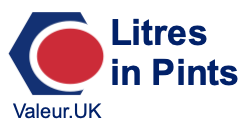3 litres in pintsThis page will show you how to convert 3 litres to pints. Before we continue, note that there is only one kind of litre, but we are referring to Imperial pints (UK pints). Therefore, when we say 3 litres in pints, we mean 3 litres (l) in UK pints (pt).

To convert 3 litres to pints, we will first make a litres to pints formula, and then enter 3 into the formula to get 3 litres in pints.

One litre is exactly 800000/454609 pints. Therefore, to convert litres to pints, we multiply litres by 800000/454609. Here is the litres to pints formula:

litres × 800000/454609 = pints

Now we can enter 3 litres into our formula to get 3 litres in pints. Here is the math, the exact fractional answer, and the approximate decimal answer to 3 litres in pints:

litres × 800000/454609 = pints
3 × 800000/454609 = pints
3 litres = 2400000/454609 pints
3 litres ≈ 5.27926 pints

Litres in Pints Converter
Please use the tool below to convert another measurement of litres to pints.

3.01 litres in pints
Here is another measurement of litres on our list that we have converted to pints for you.